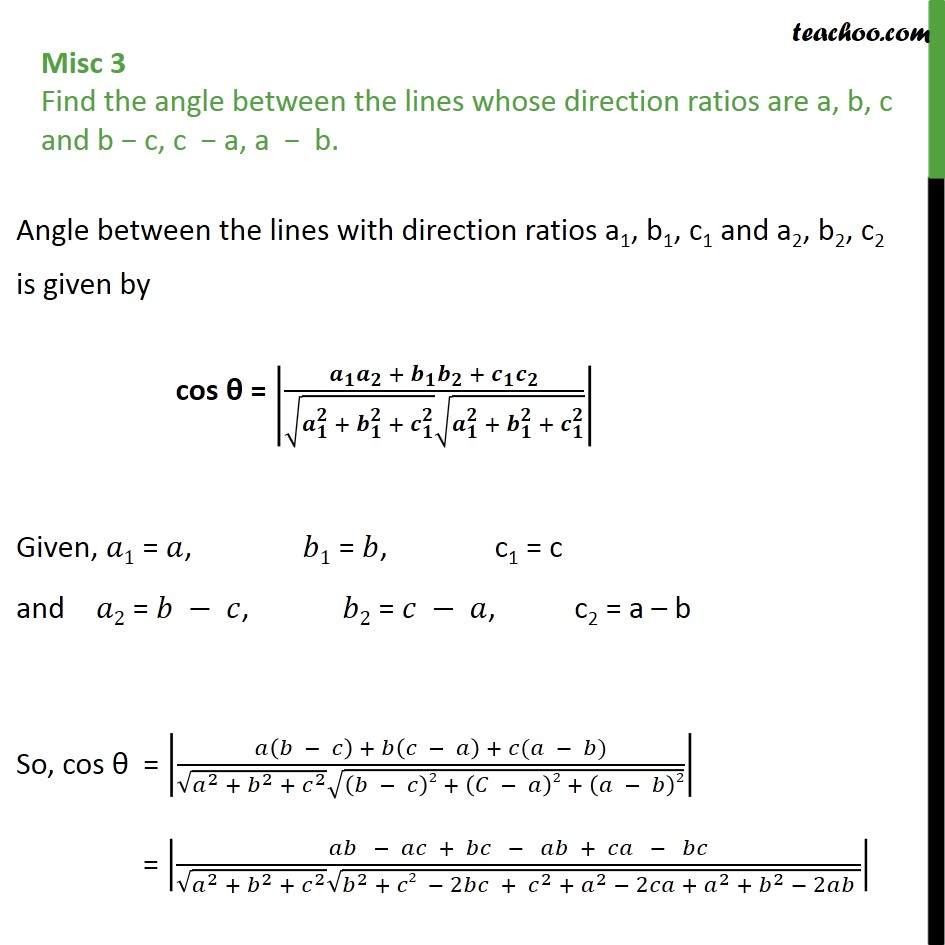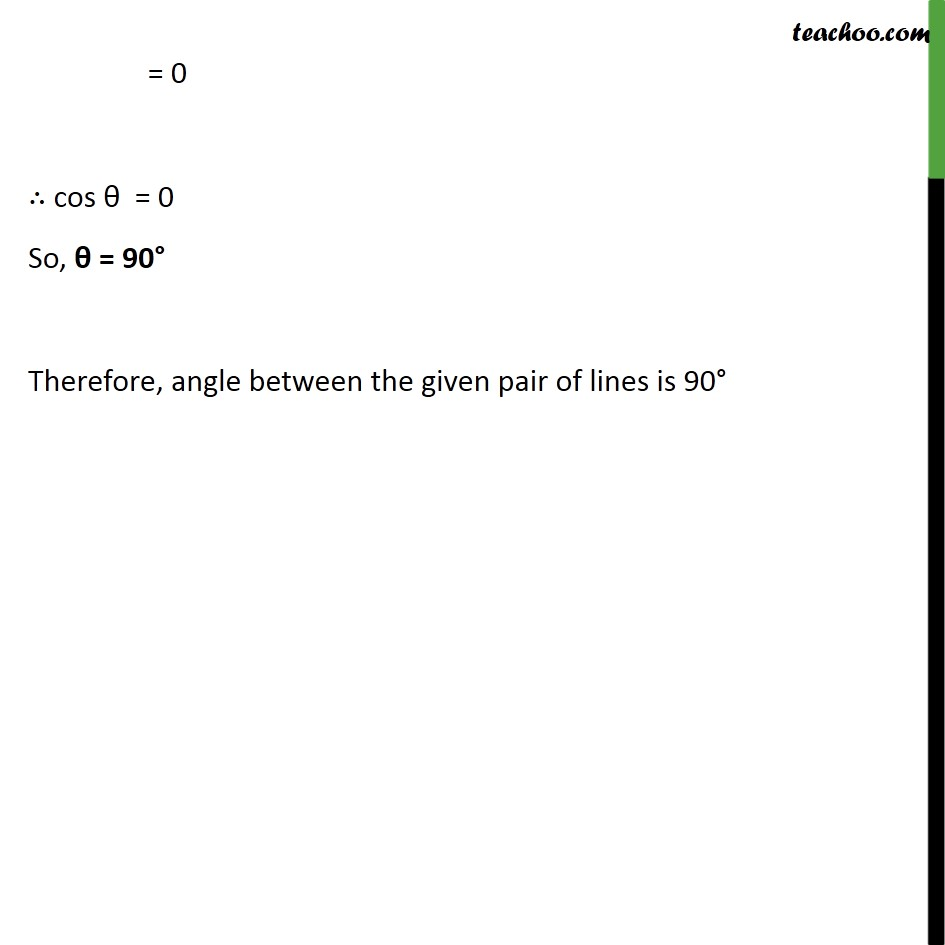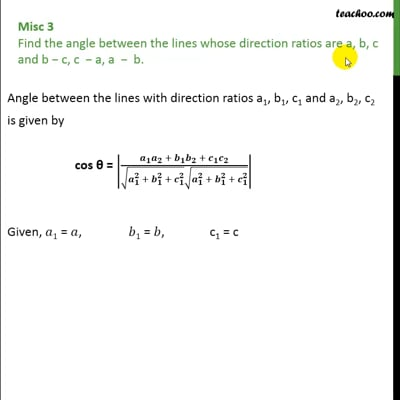Miscellaneous

Chapter 11 Class 12 Three Dimensional Geometry
Serial order wiseThis video is only available for Teachoo black users

Introducing your new favourite teacher - Teachoo Black, at only ₹83 per month

### Transcript

Misc 3 Find the angle between the lines whose direction ratios are a, b, c and b − c, c − a, a − b. Angle between the lines with direction ratios a1, b1, c1 and a2, b2, c2 is given by cos θ = 𝒂﷮𝟏﷯ 𝒂﷮𝟐﷯ + 𝒃﷮𝟏﷯ 𝒃﷮𝟐﷯ + 𝒄﷮𝟏﷯ 𝒄﷮𝟐﷯﷮ ﷮ 𝒂﷮𝟏﷮𝟐﷯ + 𝒃﷮𝟏﷮𝟐﷯ + 𝒄﷮𝟏﷮𝟐﷯﷯ ﷮ 𝒂﷮𝟏﷮𝟐﷯ + 𝒃﷮𝟏﷮𝟐﷯ + 𝒄﷮𝟏﷮𝟐﷯﷯﷯﷯ Given, 𝑎1 = 𝑎, 𝑏1 = 𝑏, c1 = c and 𝑎2 = 𝑏 − 𝑐, 𝑏2 = 𝑐 − 𝑎, c2 = a – b So, cos θ = 𝑎 𝑏 − 𝑐﷯ + 𝑏 𝑐 − 𝑎﷯ + 𝑐(𝑎 − 𝑏)﷮ ﷮ 𝑎﷮2﷯ + 𝑏﷮2﷯ + 𝑐﷮2﷯﷯ ﷮ 𝑏 − 𝑐﷯2 + 𝐶 − 𝑎﷯2 + 𝑎 − 𝑏﷯2﷯﷯﷯ = 𝑎𝑏 − 𝑎𝑐 + 𝑏𝑐 − 𝑎𝑏 + 𝑐𝑎 − 𝑏𝑐﷮ ﷮ 𝑎﷮2﷯ + 𝑏﷮2﷯ + 𝑐﷮2﷯﷯ ﷮ 𝑏﷮2﷯ + 𝑐2 − 2𝑏𝑐 + 𝑐﷮2﷯ + 𝑎﷮2﷯ − 2𝑐𝑎 + 𝑎﷮2﷯ + 𝑏﷮2﷯ − 2𝑎𝑏 ﷯﷯﷯ = 0 ∴ cos θ = 0 So, θ = 90° Therefore, angle between the given pair of lines is 90°